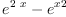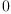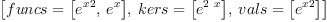login  home  contents  what's new  discussion  bug reports help  links  subscribe  changes  refresh  edit

In FriCAS expression are represented as rational functions in kernels. exp(2*x) and exp(x) are different kernels, so

fricas
exp(2*x) - exp(x)^2(1)
Type: Expression(Integer)

gives nonzero result. To recognize that this is really 0 we need to perform extra computation. In this case normalize is enough

fricas
normalize(exp(2*x) - exp(x)^2)(2)
Type: Expression(Integer)

In general, to express function in terms of algebraically independent kernels one can use rischNormalize. It takes two arguments, the first argument is list of expressions to analize, the second is list of variables (rischNormalize treats variables and constants in slightly different way). For example:

fricas
rischNormalize([exp(2*x), exp(x)],[x])(3)
Type: Record(funcs: List(Expression(Integer)),kers: List(Kernel(Expression(Integer))),vals: List(Expression(Integer)))

The result has three parts. First is list of rewritten expression. Second is list of kernels that were rewritten. The third is list of values assigned to kernels.

 Subject:   Be Bold !! ( 14 subscribers )